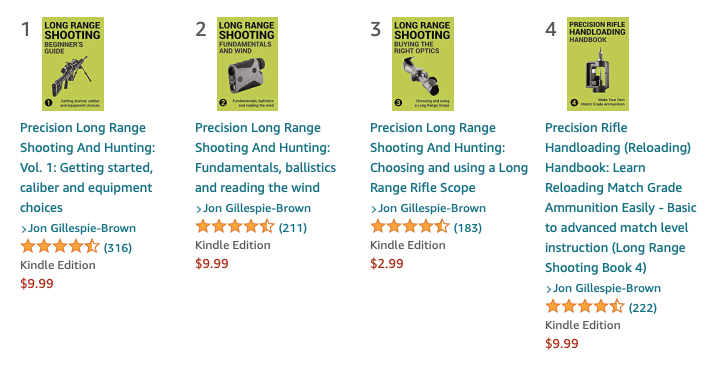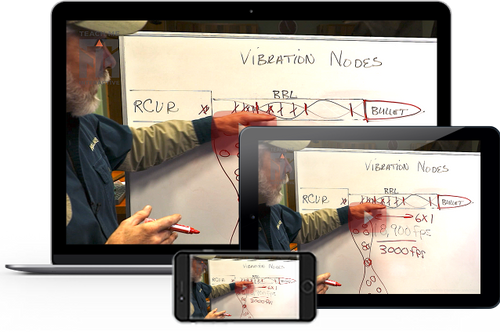# MOA and MILS explained

A MIL or an MOA linear equivalent increases proportionally with distance. With the MOA and MIL systems, you correct the position of the reticle inside the scope, based on wind deflection and trajectory of the bullet at the target’s distance.

We use an angular measure, as this is applicable to the flight path of a bullet. Angles are independent of other units of measure, and therefore simple formulas can be memorized and used by the shooter in ballistic drop tables and wind calculations.

The MOA (minute of angle) measurement system is the most popular among shooters in the United States by a wide margin.

### MOA

There are 360 degrees in a circle and each degree is divided into 60 minutes. Hence “minutes” of angle is a circle broken into increments of minutes (total: 21,600 minutes). If we round to the nearest hundredth of an inch, at 100 yards 1 degree measures 62.83 inches. One MOA, 1⁄60th of that, measures 1.047 inches. This is usually rounded down to 1.04 to simplify the math.

A minute of angle, usually used as a measure of group size, target size or shot dispersion, spans 1.047 inch at 100 yards but we round down to 1”. To calculate MOA at any distance, multiply 1.047 by the distance in yards and divide by 100.

There's another measure known as Shooter's MOA (SMOA)--rounding down MOA's true measure of 1.047 inches at 100 yards, to a much-simpler-to-calculate 1 inch at 100 yards. This is fine for short distances, but at 1,000 yards, SMOA is too inaccurate, as it's not 10 inches but 10.5 inches.

For simplicity, using SMOA here, a single minute roughly equates to 1 inch at 100 yards, so shooters get used to thinking of MOA in terms of inches.  2 MOA at 100 yards is 2 inches, 1 MOA at 400 yards is 4 inches, and so on. It's great to use angles because, as you can see, they increase proportionally with distance.

As long as the shooter sticks with 100-yard increments, the math is pretty simple to do in your head.

MOA scopes are often adjustable by 1/4 (0.25) MOA increments (approx. 1/4 inch at 100 yards).

You will hear the term MOA used a lot in long range shooting. MOA is often used to describe the size of targets, so a target at 500 yards that is 2 MOA in size (usually width), means that the target is 10 inches wide. 1 MOA at 500 yards would be 5 inches, therefore 2 MOA would be 10 (2x5) inches. However, if you are using an MOA reticle in your scope, you will use MOA as a measurement and not inches, and you will use the markings (subtension) in the scope reticle that size up targets or make adjustments. The same goes for a MIL scope reticle.

MILs, or milliradians, are a unit of measurement dividing radians in a circle. A radian is equal to 57.3 degrees, with 6.2832 (π x 2) radians in a circle. There are 1000 milliradians in 1 radian, and therefore 6,283 milliradians (or mils) in a circle. Thus, 1 MIL at 100 yards is equal to 3.6” inches and 1 MIL at 100 meters is equal to 10 centimeters. At 100 meters, 1⁄10 of a mil equals .9999 centimeter. Practically speaking, 1⁄10 of a mil equals 1 centimeter at 100 meters.

A mil is so large that it's usually broken into tenths in order to make precise adjustments on your scope turret.

So 1 mil equals 1 yard at 1,000 yards, and 1 meter at 1,000 meters.

It doesn't matter if you use metric or U.S. Scale, and that's its beauty.

Milliradian scopes are often adjustable by 1/10th (0.1) mil increments. At 100 yards, a 0.1 mil click is 0.36 inch, and a full mil is 3.6 inches (Practically speaking, 1⁄10 of a mil equals 1 centimeter at 100 meters).

MILs, like MOA, is an angular measure, and the length it represents, increases proportionally with distance. For example, 1 mil at 100 yards equals 3.6 inches, and 7.2 inches at 200 yards.

### Comparing MILs and MOA

There are 21,600 MOA in a circle, so a little quick division determines there are 3.4377 MOA per mil. At 100 yards, 3.4377 MOA equals 3.599 inches (3.4377 x 1.047). Rounded up, one mil equals 3.6 inches at 100 yards.

• To convert MILs to MOA = MULTIPLY BY 3.5 (The precise math is Mils x 3.438 = MOA)

• To convert MOA to MILs = DIVIDE BY 3.5 (The precise math is MOA / 3.438 = mils)

You may need to be able to do this conversion if you're shooting with a spotter with a MIL spotting scope while you are using a MOA rifle scope. Converting between the two can be crucial to making proper adjustments. The same is true when using off the shelf ammunition that may have data on your ammo's drop (at given yardage) in a unit of measurement your scope isn't setup for, and you don't have time to use a ballistic drop table to switch to your reticle and turret type.

### Subtensions and turrets

Subtension, the measurement of a section that a reticle covers at a certain range, is also important, especially when trying to estimate range or calculate for wind or trajectory by holding off the target. Like MILs and MOA, subtension is an angular measurement.

What you need to watch for,is a mix of different reticles and turrets, in terms of MILs and MOA. For example, you are shooting with a mil-dot reticle (one that uses MIL dots as measurements) and a MOA scope with 1⁄4-MOA clicks. Here, you might need to convert what you see through the scope in MIL measurements into MOA clicks (on the scope turrets) - this means doing math at a time when you are likely to need speed and a quick adjustment. It's hassle you don't need, so try and match the reticle to the turrets.

This is even worse if you are using a second focal plane scope that has differing measurements in the reticle, depending on the magnification you have set. Reticles in the first focal plane (FFP) maintain the same relationship to the target regardless of magnification. Reticles in the second focal plane (SFP) cause the subtension/relationship to change, as magnification is altered. Reticles set in the second focal plane are usually calibrated to work at the scope's maximum magnification (see the section in Scope for more details).  See the chapter on “choosing optics” for details on focal plane (FFP and SFP).

* * *

### Takeaway:

Once again, be aware of your mission and decide if you plan to “talk” meters/MILs or yards/MOA. Learn both systems and always be able to communicate with your fellow shooters. Be careful not to mix your reticle and turrets between either system.If you prefer to learn from videos you can find online classes and video lessons here: https://www.longrangeshootingbooks.com/Learn Long Range Shooting

Learn on your mobile device or PC with 100's of Videos, Illustrations, color Images and instructional text. Bite-sized lessons, at your own pace, anytime you want.

Read our Books and eBooks on your mobile, Tablet or eReader with 100's of Illustrations, color Images and instructional text. Buy the Series to learn the art of Long Range.

Watch hours of Instructional Videos as a compliment to our Books. Like being in class but at your convenience and without the travel. Easy to understand lessons by experts.